# Tourist

Tourist walk at 4.1 km/h. Every 5 km have 20-minute break. How many kilometers passed, when started at 4:40 and finished at 10:30?

Result

x =  19.8 km

#### Solution: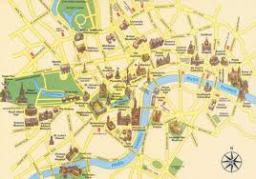Our examples were largely sent or created by pupils and students themselves. Therefore, we would be pleased if you could send us any errors you found, spelling mistakes, or rephasing the example. Thank you!

Leave us a comment of this math problem and its solution (i.e. if it is still somewhat unclear...):Be the first to comment!Tips to related online calculators
Do you have a linear equation or system of equations and looking for its solution? Or do you have quadratic equation?
Do you want to convert length units?
Do you want to convert time units like minutes to seconds?

## Next similar math problems:

1. HoursThe lesson lasts 45 minutes. For the week, students have 18 lesson hours. How many are the actual hours?
2. Family 8Father is 38 years old, daughter 12, son 14. How many years will father have as many years as his children together?
3. Three paintersOne painter would have painted the fence for 15 hours, the second in 12 hours. The plot had to be painted in four hours, so they called the third one, and all worked together. For what time would the third painter paint fence the fence alone?
4. FamilyThe twins Nina and Ema have five years younger brother Michal. Together have 43 years. How old is Michal?
5. Forest nurseryIn the forest nursery after winter, they found that 1/10 stems died out of them. For them, they land 193 new spruces. How many spruces are in the forest nursery?
6. Class alphabet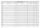All 29 pupils in the class are written in a class by alphabet. The number of pupils enrolled before Paul is three times higher than the number of pupils behind him. Calculate how many pupils are enrolled after Paul.
7. InfirmaryTwo thirds of children from the infirmary went on a trip seventh went to bathe and 40 children remained in the gym. How many children were treated in the infirmary?
8. Apartment areaAnton wanted to measure the area of the apartment. But he bought a meter only 1.5m long. Later he recalled that he had two meters long at 4.5m and 18m at home. How many times are home gauges longer than the meter he bought?
9. Father and son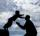Father is 44 years old, his son 16 years. Determine how many years ago was the father five times older than the son.
10. How oldThe student who asked how many years he answered: "After 10 years I will be twice as old than as I was four years ago. How old is student?
11. Bean bag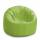A student tossed a bean bag. It landed 216 inches away. How many yards are equal to 216 inches?
12. The math testThe math test contains 20 problems. For each correctly solved problem, the solver gets 3 points, for each incorrectly solved or unsolved problem, 2 points are deducted. Ondrej got 25 points. How many problems did he solve correctly?
13. Simple equation 8Solve the following equation: 36=-(1+7x)-6(-7-x)
14. Simple equation 1035= 7*3*x what is x?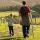Daddy is a 46 year old and son is at age of 16. When (which year) was/will daddy 5 times more years than his son?Which equation calculates the number of 1/3-foot pieces that can be cut from a piece of wood that is 7 feet long?Susan's age will be after 12 years four times as much as twelve years ago. How old is Susan now?